## 2012年1月7日 星期六

### [ The python tutorial ] 5. Data Structures

Data Structures :

- More on Lists

list.append(x)

list.extend(L)

list.insert(i, x)

list.remove(x)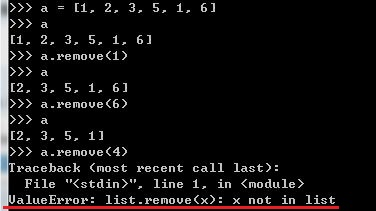list.pop([i])

list.index(x)

list.count(x)

list.sort()

list.reverse()>>> from collections import deque
>>> queue = deque(["Eric", "John", "Michael"])
>>> queue.append("Terry") # Terry arrives
>>> queue.append("Graham") # Graham arrives
>>> queue.popleft() # The first to arrive now leaves
'Eric'
>>> queue.popleft() # The second to arrive now leaves
'John'
>>> queue # Remaining queue in order of arrival
deque(['Michael', 'Terry', 'Graham'])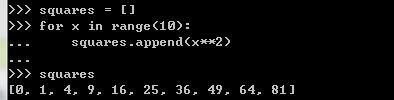>>> squares2 = [ x**2 for x in range(10)]
>>> squares2
[0, 1, 4, 9, 16, 25, 36, 49, 64, 81]>>> [(x, y) for x in [1, 2, 3] for y in [3, 1, 4] if x != y]
[(1, 3), (1, 4), (2, 3), (2, 1), (2, 4), (3, 1), (3, 4)]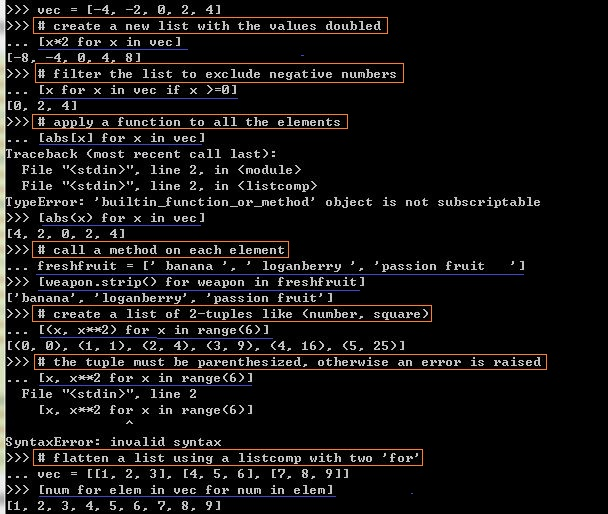>>> from math import pi
>>> [str(round(pi, i)) for i in range(1, 6)]
['3.1', '3.14', '3.142', '3.1416', '3.14159']

>>> matrix = [
... [1, 2, 3, 4],
... [5, 6, 7, 8],
... [9, 10, 11, 12],
... ]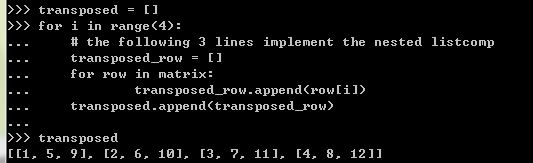>>> list(zip(*matrix))
[(1, 5, 9), (2, 6, 10), (3, 7, 11), (4, 8, 12)]

The del statement :Tuples and Sequences :

>>> t = 12345, 54321, 'hello!'
>>> t
12345
>>> t
(12345, 54321, 'hello!')
>>> # Tuples may be nested:
... u = t, (1, 2, 3, 4, 5)
>>> u
((12345, 54321, 'hello!'), (1, 2, 3, 4, 5))

tuple 與 字串都是 immutable, 也就是你不能改變 tuple 內的元素. 另外在建立空的 tuple 或是 只含一個元素的 tuple 可以如下 (只有一個元素的 tuple 後面須跟著一個逗點, 說明他是 tuple!) :
>>> empty = ()
>>> singleton = 'hello', # <-- note trailing comma
>>> len(empty)
0
>>> len(singleton)
1
>>> singleton
('hello',)Sets :
Set 為一資料結構其中的元素不允許重複, 你可以使用 mathematical operations 像 Union, Intersection, Difference, 與 Symmetric difference 來操作它. 要建立 Set 可以使用 {} 包含元素或是使用函式 set(). 但是要注意如果要建立空 Set, 就只能使用 函式 set() 而不能使用 {} (裡面沒有元素), 後者會被當作 字典 物件, 是下一節要講的東西. 底下為 Set 物件使用範例 :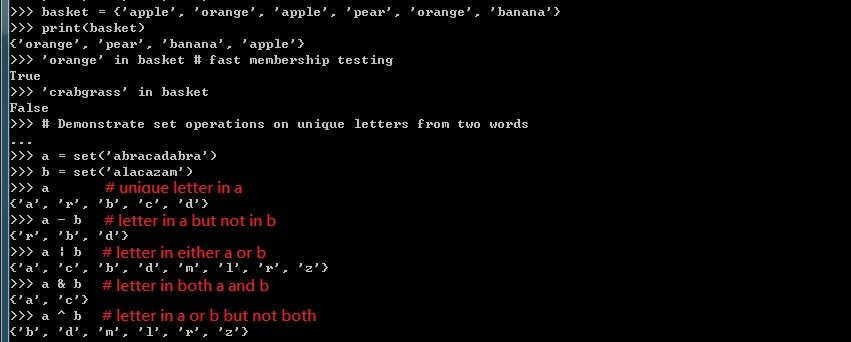>>> a = {x for x in 'abraccadabra' if x not in 'abc'}
>>> a
{'r', 'd'}

Dictionaries :>>> dict([('sape', 4139), ('guido', 4127), ('john', 4098)])
{'sape': 4139, 'john': 4098, 'guido': 4127}

>>> {x: x**2 for x in (2, 4, 6)}
{2: 4, 4: 16, 6: 36}

>>> dict(sape=4139, guido=4127, jack=4098)
{'sape': 4139, 'jack': 4098, 'guido': 4127}

Looping Techniques :

>>> knights = {'gallahad': 'the pure', 'robin': 'the brave'}
>>> for k, v in knights.items():
... print(k, v)
...
robin the brave

>>> for i, v in enumerate(['tic', 'tac', 'toe']):
... print(i, v)
...
0 tic
1 tac
2 toe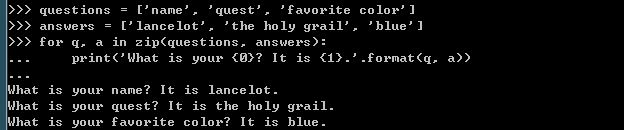>>> for i in reversed(range(1, 10, 2)):
... print(i)
...
9
7
5
3
1More on Conditions :

>>> string1, string2, string3 = '', 'John', 'Peter'
>>> non_null = string1 or string2 or string3 # '' means false.
>>> non_null # Because of shot-cut, string3 won't be assigned to non_null
'John'

Comparing Sequences and Other Types :

(1, 2, 3) < (1, 2, 4# 因為 item3 : 3<4
[1, 2, 3] < [1, 2, 4# 因為 item3 : 3<4
'ABC' < 'C' < 'Pascal' < 'Python' # 因為 'A' < 'C' , 'C' < 'P', 'a' < 'y'
(1, 2, 3, 4) < (1, 2, 4# 因為 item3 : 3<4
(1, 2) < (1, 2, -1# 因為 串列 右邊元素多
(1, 2, 3) == (1.0, 2.0, 3.0) # 值相等
(1, 2, ('aa', 'ab')) < (1, 2, ('abc', 'a'), 4) # 因為 'aa' < 'abc'

Note that comparing objects of different types with < or > is legal provided that the objects have appropriate comparison methods. For example, mixed numeric types are compared according to their numeric value, so 0 equals 0.0, etc. Otherwise, rather than providing an arbitrary ordering, the interpreter will raise aTypeError exception.
This message was edited 52 times. Last update was at 07/01/2012 18:39:24

### [ Py DS ] Ch5 - Machine Learning (Part2)

Source From  Here   Introducing Scikit-Learn   There are several Python libraries that provide solid implementations of a range of machin...